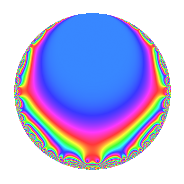# Properties

 Label 189.2.h.aLevel 189 Weight 2 Character orbit 189.h Analytic conductor 1.509 Analytic rank 0 Dimension 2 CM No Inner twists 2

# Learn more about

## Newspace parameters

 Level: $$N$$ = $$189 = 3^{3} \cdot 7$$ Weight: $$k$$ = $$2$$ Character orbit: $$[\chi]$$ = 189.h (of order $$3$$ and degree $$2$$)

## Newform invariants

 Self dual: No Analytic conductor: $$1.5091725982$$ Analytic rank: $$0$$ Dimension: $$2$$ Coefficient field: $$\Q(\sqrt{-3})$$ Coefficient ring: $$\Z[a_1, \ldots, a_{5}]$$ Coefficient ring index: $$1$$ Sato-Tate group: $\mathrm{SU}(2)[C_{3}]$

## $q$-expansion

Coefficients of the $$q$$-expansion are expressed in terms of a primitive root of unity $$\zeta_{6}$$. We also show the integral $$q$$-expansion of the trace form.

 $$f(q)$$ $$=$$ $$q$$ $$- q^{2}$$ $$- q^{4}$$ $$+ ( -1 + \zeta_{6} ) q^{5}$$ $$+ ( 3 - 2 \zeta_{6} ) q^{7}$$ $$+ 3 q^{8}$$ $$+O(q^{10})$$ $$q$$ $$- q^{2}$$ $$- q^{4}$$ $$+ ( -1 + \zeta_{6} ) q^{5}$$ $$+ ( 3 - 2 \zeta_{6} ) q^{7}$$ $$+ 3 q^{8}$$ $$+ ( 1 - \zeta_{6} ) q^{10}$$ $$+ 5 \zeta_{6} q^{11}$$ $$+ 5 \zeta_{6} q^{13}$$ $$+ ( -3 + 2 \zeta_{6} ) q^{14}$$ $$- q^{16}$$ $$+ ( 3 - 3 \zeta_{6} ) q^{17}$$ $$-\zeta_{6} q^{19}$$ $$+ ( 1 - \zeta_{6} ) q^{20}$$ $$-5 \zeta_{6} q^{22}$$ $$+ ( 3 - 3 \zeta_{6} ) q^{23}$$ $$+ 4 \zeta_{6} q^{25}$$ $$-5 \zeta_{6} q^{26}$$ $$+ ( -3 + 2 \zeta_{6} ) q^{28}$$ $$+ ( -1 + \zeta_{6} ) q^{29}$$ $$-5 q^{32}$$ $$+ ( -3 + 3 \zeta_{6} ) q^{34}$$ $$+ ( -1 + 3 \zeta_{6} ) q^{35}$$ $$-3 \zeta_{6} q^{37}$$ $$+ \zeta_{6} q^{38}$$ $$+ ( -3 + 3 \zeta_{6} ) q^{40}$$ $$-5 \zeta_{6} q^{41}$$ $$+ ( 1 - \zeta_{6} ) q^{43}$$ $$-5 \zeta_{6} q^{44}$$ $$+ ( -3 + 3 \zeta_{6} ) q^{46}$$ $$+ ( 5 - 8 \zeta_{6} ) q^{49}$$ $$-4 \zeta_{6} q^{50}$$ $$-5 \zeta_{6} q^{52}$$ $$+ ( -9 + 9 \zeta_{6} ) q^{53}$$ $$-5 q^{55}$$ $$+ ( 9 - 6 \zeta_{6} ) q^{56}$$ $$+ ( 1 - \zeta_{6} ) q^{58}$$ $$-14 q^{61}$$ $$+ 7 q^{64}$$ $$-5 q^{65}$$ $$+ 4 q^{67}$$ $$+ ( -3 + 3 \zeta_{6} ) q^{68}$$ $$+ ( 1 - 3 \zeta_{6} ) q^{70}$$ $$+ 12 q^{71}$$ $$+ ( -3 + 3 \zeta_{6} ) q^{73}$$ $$+ 3 \zeta_{6} q^{74}$$ $$+ \zeta_{6} q^{76}$$ $$+ ( 10 + 5 \zeta_{6} ) q^{77}$$ $$+ 8 q^{79}$$ $$+ ( 1 - \zeta_{6} ) q^{80}$$ $$+ 5 \zeta_{6} q^{82}$$ $$+ ( -9 + 9 \zeta_{6} ) q^{83}$$ $$+ 3 \zeta_{6} q^{85}$$ $$+ ( -1 + \zeta_{6} ) q^{86}$$ $$+ 15 \zeta_{6} q^{88}$$ $$-13 \zeta_{6} q^{89}$$ $$+ ( 10 + 5 \zeta_{6} ) q^{91}$$ $$+ ( -3 + 3 \zeta_{6} ) q^{92}$$ $$+ q^{95}$$ $$+ ( 9 - 9 \zeta_{6} ) q^{97}$$ $$+ ( -5 + 8 \zeta_{6} ) q^{98}$$ $$+O(q^{100})$$ $$\operatorname{Tr}(f)(q)$$ $$=$$ $$2q$$ $$\mathstrut -\mathstrut 2q^{2}$$ $$\mathstrut -\mathstrut 2q^{4}$$ $$\mathstrut -\mathstrut q^{5}$$ $$\mathstrut +\mathstrut 4q^{7}$$ $$\mathstrut +\mathstrut 6q^{8}$$ $$\mathstrut +\mathstrut O(q^{10})$$ $$2q$$ $$\mathstrut -\mathstrut 2q^{2}$$ $$\mathstrut -\mathstrut 2q^{4}$$ $$\mathstrut -\mathstrut q^{5}$$ $$\mathstrut +\mathstrut 4q^{7}$$ $$\mathstrut +\mathstrut 6q^{8}$$ $$\mathstrut +\mathstrut q^{10}$$ $$\mathstrut +\mathstrut 5q^{11}$$ $$\mathstrut +\mathstrut 5q^{13}$$ $$\mathstrut -\mathstrut 4q^{14}$$ $$\mathstrut -\mathstrut 2q^{16}$$ $$\mathstrut +\mathstrut 3q^{17}$$ $$\mathstrut -\mathstrut q^{19}$$ $$\mathstrut +\mathstrut q^{20}$$ $$\mathstrut -\mathstrut 5q^{22}$$ $$\mathstrut +\mathstrut 3q^{23}$$ $$\mathstrut +\mathstrut 4q^{25}$$ $$\mathstrut -\mathstrut 5q^{26}$$ $$\mathstrut -\mathstrut 4q^{28}$$ $$\mathstrut -\mathstrut q^{29}$$ $$\mathstrut -\mathstrut 10q^{32}$$ $$\mathstrut -\mathstrut 3q^{34}$$ $$\mathstrut +\mathstrut q^{35}$$ $$\mathstrut -\mathstrut 3q^{37}$$ $$\mathstrut +\mathstrut q^{38}$$ $$\mathstrut -\mathstrut 3q^{40}$$ $$\mathstrut -\mathstrut 5q^{41}$$ $$\mathstrut +\mathstrut q^{43}$$ $$\mathstrut -\mathstrut 5q^{44}$$ $$\mathstrut -\mathstrut 3q^{46}$$ $$\mathstrut +\mathstrut 2q^{49}$$ $$\mathstrut -\mathstrut 4q^{50}$$ $$\mathstrut -\mathstrut 5q^{52}$$ $$\mathstrut -\mathstrut 9q^{53}$$ $$\mathstrut -\mathstrut 10q^{55}$$ $$\mathstrut +\mathstrut 12q^{56}$$ $$\mathstrut +\mathstrut q^{58}$$ $$\mathstrut -\mathstrut 28q^{61}$$ $$\mathstrut +\mathstrut 14q^{64}$$ $$\mathstrut -\mathstrut 10q^{65}$$ $$\mathstrut +\mathstrut 8q^{67}$$ $$\mathstrut -\mathstrut 3q^{68}$$ $$\mathstrut -\mathstrut q^{70}$$ $$\mathstrut +\mathstrut 24q^{71}$$ $$\mathstrut -\mathstrut 3q^{73}$$ $$\mathstrut +\mathstrut 3q^{74}$$ $$\mathstrut +\mathstrut q^{76}$$ $$\mathstrut +\mathstrut 25q^{77}$$ $$\mathstrut +\mathstrut 16q^{79}$$ $$\mathstrut +\mathstrut q^{80}$$ $$\mathstrut +\mathstrut 5q^{82}$$ $$\mathstrut -\mathstrut 9q^{83}$$ $$\mathstrut +\mathstrut 3q^{85}$$ $$\mathstrut -\mathstrut q^{86}$$ $$\mathstrut +\mathstrut 15q^{88}$$ $$\mathstrut -\mathstrut 13q^{89}$$ $$\mathstrut +\mathstrut 25q^{91}$$ $$\mathstrut -\mathstrut 3q^{92}$$ $$\mathstrut +\mathstrut 2q^{95}$$ $$\mathstrut +\mathstrut 9q^{97}$$ $$\mathstrut -\mathstrut 2q^{98}$$ $$\mathstrut +\mathstrut O(q^{100})$$

## Character Values

We give the values of $$\chi$$ on generators for $$\left(\mathbb{Z}/189\mathbb{Z}\right)^\times$$.

 $$n$$ $$29$$ $$136$$ $$\chi(n)$$ $$-\zeta_{6}$$ $$-\zeta_{6}$$

## Embeddings

For each embedding $$\iota_m$$ of the coefficient field, the values $$\iota_m(a_n)$$ are shown below.

For more information on an embedded modular form you can click on its label.

Label $$\iota_m(\nu)$$ $$a_{2}$$ $$a_{3}$$ $$a_{4}$$ $$a_{5}$$ $$a_{6}$$ $$a_{7}$$ $$a_{8}$$ $$a_{9}$$ $$a_{10}$$
37.1
 0.5 − 0.866025i 0.5 + 0.866025i
−1.00000 0 −1.00000 −0.500000 0.866025i 0 2.00000 + 1.73205i 3.00000 0 0.500000 + 0.866025i
46.1 −1.00000 0 −1.00000 −0.500000 + 0.866025i 0 2.00000 1.73205i 3.00000 0 0.500000 0.866025i
 $$n$$: e.g. 2-40 or 990-1000 Significant digits: Format: Complex embeddings Normalized embeddings Satake parameters Satake angles

## Inner twists

Char. orbit Parity Mult. Self Twist Proved
1.a Even 1 trivial yes
63.h Even 1 no

## Hecke kernels

This newform can be constructed as the kernel of the linear operator $$T_{2}$$ $$\mathstrut +\mathstrut 1$$ acting on $$S_{2}^{\mathrm{new}}(189, [\chi])$$.